# 1762 Happy Saint Patrick’s Day!

### Today’s ♣ Puzzle:

Here’s a much easier puzzle than yesterday’s for you to enjoy on this Saint Patrick’s Day. The diagonal lines on the corner boxes are only to help define the leaves of the shamrock.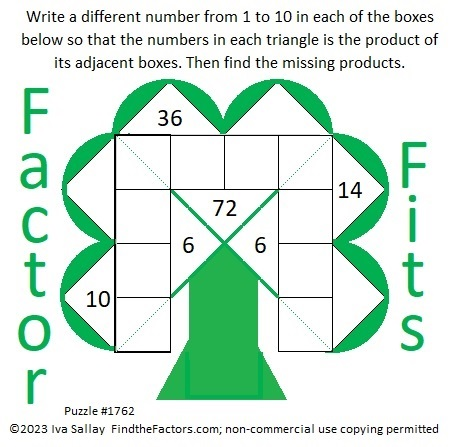### Factors of 1762:

• 1762 is a composite number.
• Prime factorization: 1762 = 2 × 881.
• 1762 has no exponents greater than 1 in its prime factorization, so √1762 cannot be simplified.
• The exponents in the prime factorization are 1 and 1. Adding one to each exponent and multiplying we get (1 + 1)(1 + 1) = 2 × 2 = 4. Therefore 1762 has exactly 4 factors.
• The factors of 1762 are outlined with their factor pair partners in the graphic below.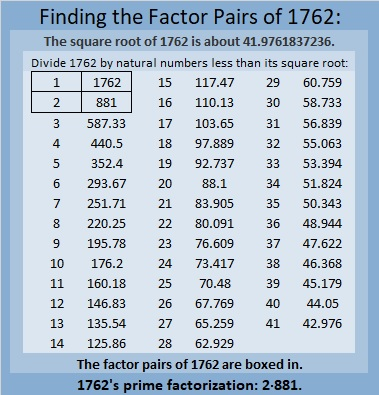### More About the Number 1762:

1762 is the sum of two squares:
41² + 9² = 1762.

1762 is the hypotenuse of a Pythagorean triple:
738-1600-1762 calculated from 2(41)(9), 41² – 9², 41² + 9².
It is also 2 times (369-800-881).

1762 is palindrome 7C7 in base 15
because 7(15²) + 12(15) + 7(1) = 1762.

# 1749 Happy Valentine’s Day!

### Today’s Puzzle:

Here’s one more Valentine’s puzzle for you to do. Enjoy!### Factors of 1749:

1749 is divisible by 3 because 7 is half of 14 so any 3-digit number made from the digits 714 in any order is divisible by 3, and of course, 9 is divisible by 3.

1749 is divisible by 11 because 1 – 7 + 4 – 9 = -11, a multiple of 11.

• 1749 is a composite number.
• Prime factorization: 1749 = 3 × 11 × 53.
• 1749 has no exponents greater than 1 in its prime factorization, so √1749 cannot be simplified.
• The exponents in the prime factorization are 1, 1, and 1. Adding one to each exponent and multiplying we get (1 + 1)(1 + 1)(1 + 1) = 2 × 2 × 2 = 8. Therefore 1749 has exactly 8 factors.
• The factors of 1749 are outlined with their factor pair partners in the graphic below.### More About the Number 1749:

1749 is the hypotenuse of a Pythagorean triple:
924-1485-1749 which is 33 times (28-45-53).

1749 is the difference of two squares in FOUR different ways:
875² – 874² = 1749,
293² – 290² = 1749,
85² – 74² = 1749, and
43² – 10² = 1749.

# 1748 A Factor Fits Valentine’s Puzzle

### Today’s Puzzle:

Can you find the one and only solution to this Factor Fits Valentine puzzle or will it give you fits?### Factors of 1748:

• 1748 is a composite number.
• Prime factorization: 1748 = 2 × 2 × 19 × 23, which can be written 1748 = 2² × 19 × 23.
• 1748 has at least one exponent greater than 1 in its prime factorization so √1748 can be simplified. Taking the factor pair from the factor pair table below with the largest square number factor, we get √1748 = (√4)(√437) = 2√437.
• The exponents in the prime factorization are 2, 1, and 1. Adding one to each exponent and multiplying we get (2 + 1)(1 + 1)(1 + 1) = 3 × 2 × 2 = 12. Therefore 1748 has exactly 12 factors.
• The factors of 1748 are outlined with their factor pair partners in the graphic below.### More About the Number 1748:

1748 is the difference of two squares in two ways:
438² – 436² = 1748, and
42² – 4² = 1748.
That means we are only 16 numbers away from the next perfect square!

# 1717 Factor Fits for Your Valentine

### Today’s Puzzle:

Solve this Valentine-themed Factor Fits puzzle with both logic and heart!### Factors of 1717:

• 1717 is a composite number.
• Prime factorization: 1717 = 17 × 101.
• 1717 has no exponents greater than 1 in its prime factorization, so √1717 cannot be simplified.
• The exponents in the prime factorization are 1 and 1. Adding one to each exponent and multiplying we get (1 + 1)(1 + 1) = 2 × 2 = 4. Therefore 1717 has exactly 4 factors.
• The factors of 1717 are outlined with their factor pair partners in the graphic below.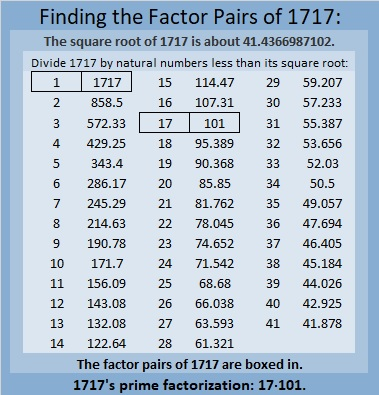### More About the Number 1717:

1717 is the sum of two squares two different ways:
41² + 6² = 1717, and
39² + 14² = 1717.

1717 is the hypotenuse of FOUR Pythagorean triples:
340 1683 1717, which is 17 times (20-99-101),
492 1645 1717, calculated from 2(41)(6), 41² – 6², 41² + 6²,
808 1515 1717, which is (8-15-17) times 101, and
1092 1325 1717, calculated from 2(39)(14), 39² – 14², 39² + 14².

# 1714 Will the Factors in This Puzzle Give You Fits?

### Today’s Puzzle:

12 and 24 have several common factors, but only one of them works in this puzzle. Will it be 2, 3, 4, 6, or 12?

What about 40 and 60’s common factors?

Don’t guess which factor to use. Start elsewhere in the puzzle where there’s only one possible common factor. Then use logic to eliminate some of the factor possibilities for 12, 24 and 40, 60. You will have to think, but it won’t be too difficult.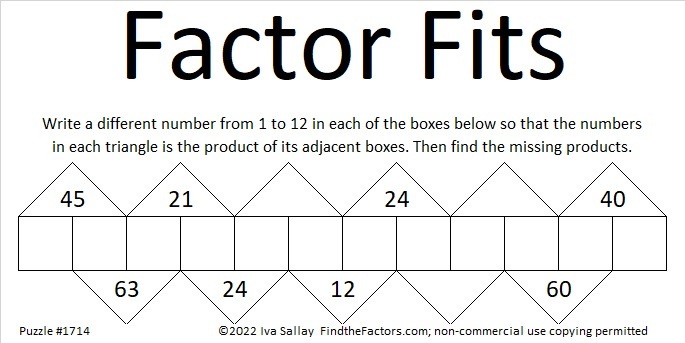### Factors of 1714:

• 1714 is a composite number.
• Prime factorization: 1714 = 2 × 857.
• 1714 has no exponents greater than 1 in its prime factorization, so √1714 cannot be simplified.
• The exponents in the prime factorization are 1 and 1. Adding one to each exponent and multiplying we get (1 + 1)(1 + 1) = 2 × 2 = 4. Therefore 1714 has exactly 4 factors.
• The factors of 1714 are outlined with their factor pair partners in the graphic below.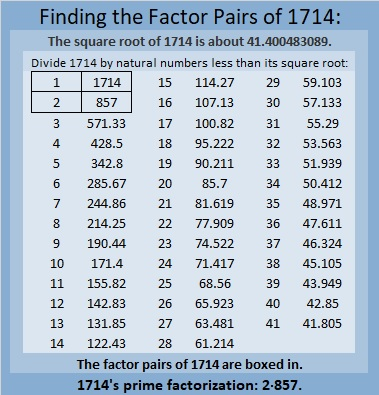### More About the Number 1714:

1714 is the sum of two squares:
33² + 25² = 1714.

1714 is the hypotenuse of a Pythagorean triple:
464-1650-1714, calculated from 33² – 25², 2(33)(25), 33² + 25².
It is also 2 times (232-825-857).

# 1712 Can You Make the Factors Fit?

### Today’s Puzzle:

This Factor Fits puzzle starts off fairly easy before it potentially might give you fits trying to place the rest of the factors. Are you game?### Factors of 1712:

• 1712 is a composite number.
• Prime factorization: 1712 = 2 × 2 × 2 × 2 × 107, which can be written 1712 = 2⁴ × 107.
• 1712 has at least one exponent greater than 1 in its prime factorization so √1712 can be simplified. Taking the factor pair from the factor pair table below with the largest square number factor, we get √1712 = (√16)(√107) = 4√107.
• The exponents in the prime factorization are 4 and 1. Adding one to each exponent and multiplying we get (4 + 1)(1 + 1) = 5 × 2 = 10. Therefore 1712 has exactly 10 factors.
• The factors of 1712 are outlined with their factor pair partners in the graphic below.### More About the Number 1712:

1712 is the difference of two squares in three different ways:
429² – 427² = 1712,
216² – 212² = 1712, and
111² – 103² = 1712.

# 1708 Happy Birthday, Jo Morgan!

### Today’s Puzzle:

A few days ago I published a new kind of factoring puzzle. Jo Morgan of Resourceaholic.com, keeps an eye out for new mathematical resources on Twitter. She was one of the first to notice and like my puzzle. Because of her, lots of other people noticed the puzzle, too. Today is Jo’s birthday, and I decided to make a similar puzzle for her to enjoy. You might find it slightly more difficult than the earlier puzzle, but use logic from the beginning, and you will be able to fit in all the factors.### Factors of 1708:

• 1708 is a composite number.
• Prime factorization: 1708 = 2 × 2 × 7 × 61, which can be written 1708 = 2² × 7 × 61.
• 1708 has at least one exponent greater than 1 in its prime factorization so √1708 can be simplified. Taking the factor pair from the factor pair table below with the largest square number factor, we get √1708 = (√4)(√427) = 2√427.
• The exponents in the prime factorization are 2, 1, and 1. Adding one to each exponent and multiplying we get (2 + 1)(1 + 1)(1 + 1) = 3 × 2 × 2 = 12. Therefore 1708 has exactly 12 factors.
• The factors of 1708 are outlined with their factor pair partners in the graphic below.### More About the Number 1708:

1708 is the hypotenuse of a Pythagorean triple:
308-1680-1708, which is 28 times (11-60-61).